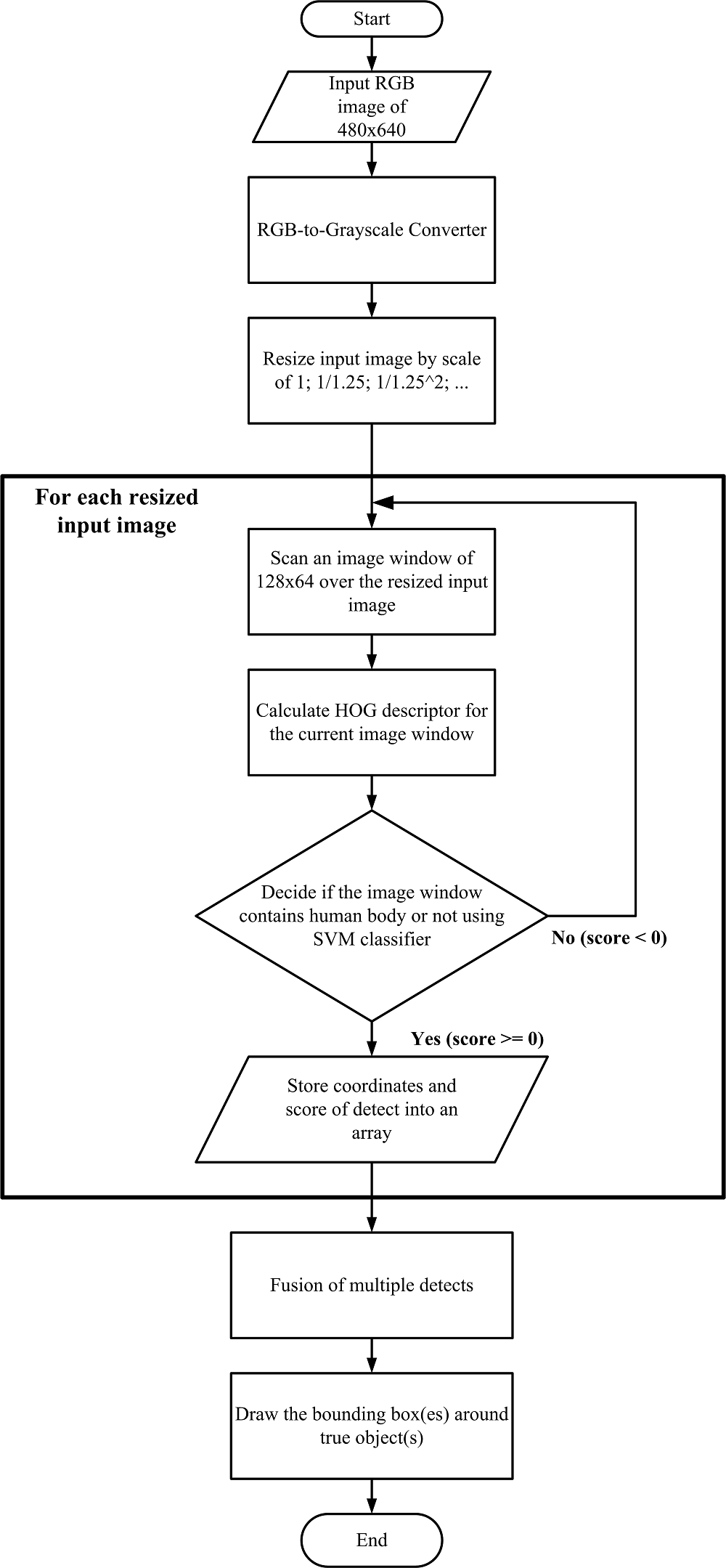# 20th LSI Design Contests・in Okinawa  Design Specification - 3-3

## 3-3. Algorithm

The algorithm of the person detection using Histogram of Oriented Gradient based on the program that was designed in MATLAB® is shown below.
In addition, it was created and verified using MATLAB®R2012a (7.14.0.739).

• zip file：En_human_detection.zip
• Image and m-file, mat-file are included in a zip file. Simulation of human detection is carried out when carry out 'humanDetector_16_09_13.m' which is this main m-file.

(1)Input image
Input the RGB image.

(2)Grayscaling and binarization
Since the detection process using a color image is difficult, the input image is converted into grayscale image. We use the NTSC Coef. method to grayscale of the image. The NTSC Coef. Method, in one method of converting from a color image to 256-level grayscale image, it can be calculated by the following equation.

Y=0.298912*R+0.586611*G+0.114478*B

Coefficients of this equation are obtained from the human visual characteristics experimentally for the color.

(3)Detection process
(3-1) Resize grayscale input image at different scales In our algorithm, the detection process uses an image window of fixed-size (128 x 64 pixels) to scan over the whole input image, and the size of the human body in each image varies at different scale. Therefore, algorithm needs to sequentially resize the original-size input image into images with smaller sizes (as long as the image window can be included in the resized input image).

(3-2) With each resized input image:

+ Scan the image window (of 128 x 64 pixels) over the image

+ At each position of scanning, compute the Histogram of Oriented Gradient (HOG) descriptor  for the image window.

+ Determine the image window to have human body or not, using the trained linear Support Vector Machine (SVM) classifier.

+ If the image window include a human body inside, save its position in the resized image (i.e. coordinates and the scaleused) and detecting score for the next step.

(4)Fusion of multiple detects
At each resized input image, there can be more than one detect around each unique human body. In other words, it is possible to have many overlapping detects over each human body in the input image. The final target is to fusing these overlapping detects so that there is only one detect for each human body.

(5)Output
Draw bounding boxes around true human bodies (if any) in the input image.

The flowchart of the algorithm is shown in Fig 4．Fig 4：Flowchart of template matching

## Reference

 機械知覚&ロボティクス研究グループ，中部大学 “局所特徴量と統計学習手法による物体検出”
http://www.slideshare.net/MPRG_Chubu_University/ss-32258845

 藤井 龍也，中島 克人，野口 祥宏，西田 健次，" HOG と SVM による上半身検出器の特徴の抽出位置に関する考察"， FIT2011（第10回情報科学技術フォーラム)，pp.105-106，2011．

http://ci.nii.ac.jp/els/110009622743.pdf?id=ART0010089832&type=pdf&lang=jp&host=cinii&order_no=&ppv_type=0&lang_sw=&no=1473992065&cp=

 INRIA Person Dataset，

http://pascal.inrialpes.fr/data/human/

 LIBLINEAR -- A Library for Large Linear Classification，

http://www.csie.ntu.edu.tw/~cjlin/liblinear/